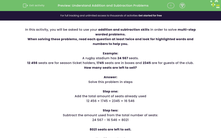# Understand Addition and Subtraction Problems

In this worksheet, students will be asked to use addition and subtraction skills in order to solve multi-step worded problems.Key stage:  KS 2

Curriculum topic:   Number: Addition and Subtraction

Curriculum subtopic:   Solve Multi-Step Add/Subtract Problems

Difficulty level:#### Worksheet Overview

In this activity, you will be asked to use your addition and subtraction skills in order to solve multi-step worded problems.

When solving these problems, read each question at least twice and look for highlighted words and numbers to help you.

Example:

A rugby stadium has 24 567 seats.

12 456 seats are for season ticket holders, 1745 seats are in boxes and 2345 are for guests of the club.

How many seats are left to sell?

Solve this problem in steps

Step one:

12 456 + 1745 + 2345 = 16 546

Step two:

Subtract the amount used from the total number of seats:

24 567 - 16 546 = 8021

8021 seats are left to sell.